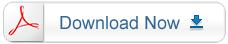#### PRGBIC-L0315-JZD-Specification F3 LED LAMP

The product nomenclature is designated as below:

X1X2 – X3X4X5X6

Designation

 X1 Package = P X2 Color U = UV B = Blue G = Green R = Red RGBIC = Red,Green,Blue with Control IC X3 Product Type L = Lamp        M=Material SMD S = SMD X4-X6 PKG Size 563=5630    314=3014    328=3528 330=3030    550=5050    320=3020 35V=3535(Vertical)    35H=3535(Horizontal)>”)}